Using Excel to Model Optimal Forest Rotation - FreeEconHelp.com, Learning Economics... Solved!

## 8/25/11

This is a highly specialized post, but I see more and more students attempting to model basic dynamic optimization problems in excel.  Here is a good example and tutorial of how to figure out optimal rotation times for a forest, a classic economics problem.

If you are having problems viewing the equations, try an up to date version of firefox, chrome, or Opera.

***Excel tips:
1) You need to type ‘=’ before an equation in a cell in excel.  Otherwise excel treats it as text, or a number, not a function.
2) If you type in ‘B1’, when you drag it down a row it will read ‘B2’, to prevent this type ‘\$B\$1’, The \$ sign means that excel will always reference the same cell, and will not automatically change it.

Sheet 1: Recreating information tables from textbooks.
This is relatively easy, we take the information from the text and program the equations into excel to replicate the table we find in the text.  However, some of the values may not match up.  See if your particular table agrees with the text or my excel spreadsheet.

Sheet 2: This sheet models the optimal rotation time of a forest stand.  It is a little more complicated, but the numbers next to NB represent the net benefit of each rotation number.  F9 shows the optimal time which is inputted into excel’s solver tool.  The G and H column stand the exogenous variables that we can change.  By clicking on each value in the D column, you can see the equation I used to represent the utility function.

For example, D1 contains: =((5000*F9^H5)*(H4-H2))*(1/(1+H3))^F9-H1

This is identical to: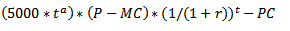Where:
t = time
a = growth (represented as an exponent)
P = price
MC = Marginal Cost
r = discount rate
PC = Planting cost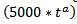= is the growth function of the forest (which is a rough comparison to the Douglas fir).

If we look at D9 it says: =((5000*F9^H5)*(H4-H2))*(1/(1+H3))^F9-H1+(((5000*F9^H5)*(H4-H2))*(1/(1+H3))^F9-H1)*(1/(1+H3))^F9+(((5000*F9^H5)*(H4-H2))*(1/(1+H3))^F9-H1)*(1/(1+H3))^(2*F9)

I know this looks complicated by it is simply D1 3 times, with an additional discount rate added in front of each additional D1 equation.

This is identical to: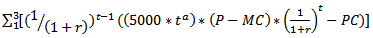This means that D10 and D11 are: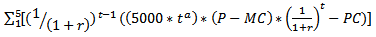And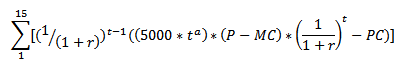Respectively.

Sheet 4: This models optimal harvest choice in each time period beginning with a stock of forest.  The problem we are faced with is deciding how many trees to cut down in each time period in order to maximize our utility.

The F and G columns show our exogenous variables, namely: The discount rate we apply to the problem, the beginning stock of the forest (or amount of trees), the exponent term in each utility function, and finally our total utility from foraging and harvesting over the time frame.
G5 and G6 show the value of the exponent.  We need this to be a value between 0 and 1 so that we satisfy the idea of diminishing marginal utility.

Columns C and D show the utility we receive from each time period from harvesting and foraging.
If you click on C2 you will see: =(E2^\$G\$6)*((1/(1+\$G\$2))^(A2-1))

This is identical to: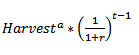And D2: =(B2^\$G\$7)*((1/(1+\$G\$2))^(A2-1))

Which is identical to: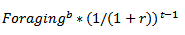Finally, the B column shows the stock of forest.  This column is equal to the remaining amount of trees left in our stand.  It begins at the value shown in G3 (500) and will go down by the amount shown in the E column for every time period.

So the B column would have the equation: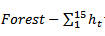for all time periods.

C17 equals the sum of C2 through C16, and D17 equals the sum of D2 through D16.  G17 equals C17 plus D17.

Ultimately, we are interested in the E column, our choice of harvest.  We use excel’s solver tool to get this.  The tool is available under the “data” command on the top part of excel.  It should be on the far right side of the options.  If you do not see it, it is probably not installed.  To install it, click on the office button (or File), and click excel options (near the bottom).  No click on “Add ins”, and click on manage add ins.  Find solver and click go or add.  It may take a minute, but then the solver tool will be added.

Open the solver tool, we want the target cell to be G17, we want to maximize this.  The “By changing cells” are out choices, or column E2 through E16.  We also need to add in constraints, such as harvest and forest always being greater than zero.  Add in these constraints and click solve and it should populate column E with values that maximize G17.  You have now just solved the model!!!

1.Anonymous26 November

Great example, but I can't find the link to the excel sheet?

2.Reason for existing is synonymous with inspiration in this unique situation.CENTRAL KENYAThe more prominent one's wants or objectives towards accomplishing a specific result are, the more noteworthy the reason.

3.I feel really happy to have seen your webpage and look forward to so many more entertaining times reading here. sherwood hall schoolThanks once more for all the details.

4.NLP and AI can help prevent school shootings. For example, Columbia University researchers have processed 2 million tweets posted by 9,000 at-risk youth to determine how language changes as a teen gets closer and closer to committing a violent act.https://www.techpally.com/how-natural-language-processing-works/

5.This content material is written tremendously dexterously. Your use of formatting gone making your points makes your rationalization completely sure and easy to take. thank you. Advanced Excel Training Mumbai

6.Thanks a lot for this affordable papers reviews
awesome post. Keep up the good work. I’ll be coming back lots. I agree with most of the points you make within this content.